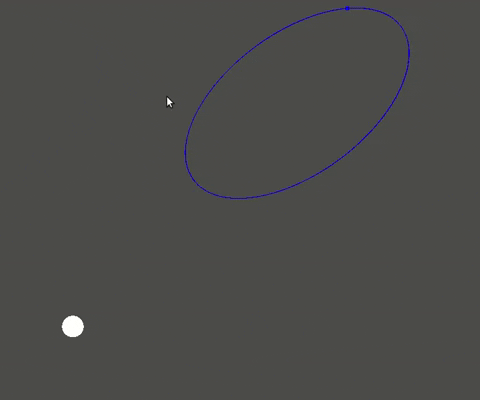I have a simple 2D demo of a dot orbiting a larger circle. This dot moves by iterating on an array of previously calculated coordinates (Vector2).

I tried to draw a line along the coordinates of this array, but the resulting line is not being drawn along the path the dot is following, even though the coordinates are the same. There is a gif bellow to ilustrate, and the code for drawing the line and moving the dot.

How can i draw this path correctly?``````extends KinematicBody2D

func _process(delta):
while len(self.path) < 1000:
var step = self.path.back()
self.path.push_back(step_movement(step))

var step = self.path.pop_front()
self.position = step.p
update()

func _draw():
draw_circle(position, size, color)

var varray = PoolVector2Array()
for step in self.path:
varray.push_back(step.p)
draw_polyline(varray, color)
``````
Godot version 3.2.1
in Engine

The polyline function you're using is inheriting the position of the circle. As the circle orbits, the entire orbit path orbits with it. If you separate the orbit line into a different sibling object that isn't parented to the KinematicBody2D that governs the circle's position, it shouldn't move when the circle moves.
Example scene tree:

-Node
|-Kinematic2D to represent the object orbiting
|-Separate object (maybe a Line2D) to represent the orbital path

I would put the data about the orbit in the parent Node, and make the children ask for it when `_ready()` or if the orbit changes.

by (674 points)
selected by

Thanks! I will make the changes you suggested